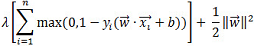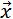# 1.1 - 8.10 - SVMDense Syntax Elements - Teradata Vantage

## Teradata Vantage™ - Machine Learning Engine Analytic Function Reference

Product
Release Number
1.1
8.10
Published
October 2019
Content Type
Programming Reference
Publication ID
B700-4003-079K
Language
English (United States)
ModelTable
Specify the name of the model table (which must not exist).
IDColumn
Specify the name of the InputTable column that contains the identifiers of the training samples.
TargetColumns
Specify the names of the InputTable columns that contain the attributes, which must have numeric data types.
KernelFunction
[Optional] Specify the kernel function that the SVMDense function uses to compute the hash function:
Option Description
'linear' (Default) SVMDense uses a Pegasos algorithm to solve linear SVM.
'polynomial' SVMDense uses a Hash-SVM algorithm.

Formula for polynomial:

γ(u T v + c) d

When SVMDense uses a Hash-SVM algorithm, each sample is represented by compact hash bits, over which an inner product is defined to serve as the surrogate of the original nonlinear kernels.

'rbf' SVMDense uses a Hash-SVM algorithm.

Formula for RBF:

exp (-γ * | | x - x' | |2)

'sigmoid' SVMDense uses a Hash-SVM algorithm.

Formula for sigmoid:

tanh (γ * u T v + c)

Gamma
[Optional] Use only when KernelFunction is 'polynomial', 'RBF', or 'sigmoid'. Specify γ in the formula. The gamma must be a positive DOUBLE value.
Default: 1.0
Constant
[Optional] Use only when KernelFunction is 'polynomial' or 'sigmoid'. Specify c in the formula. The c must be a DOUBLE value. If KernelFunction is polynomial, the minimum c value is 0.0.
Default: 1.0
Degree
[Optional] Use only when KernelFunction is 'polynomial'. Specify d in the formula. The d must be a positive INTEGER.
Default: 2
SubspaceDimension
[Optional] Use only when KernelFunction is 'polynomial', 'sigmoid', or 'rbf'. Specify the random subspace dimension of the basis matrix V obtained by the Gram-Schmidt process. The subspace_dimension must be in the range [1, 2048]. Because the Gram-Schmidt process cannot be parallelized, this dimension cannot be too large. Accuracy increases with higher subspace_dimension values, but computation costs also increase.
Default: 256
HashBits
[Optional] Use only when KernelFunction is 'polynomial', 'RBF', or 'sigmoid'. Specify the number of compact hash bits that represent a data point. The hash_bits must be in the range [8, 8192]. Accuracy increases with higher hash_bits values, but computation costs also increase.
Default: 256
ResponseColumn
Specify the name of the InputTable column that contains the class identifiers of the samples. The response_column must have an integer or string data type.
RegularizationLambda
[Optional] Specify the regularization parameter λ in the SVM soft-margin loss function:The lambda must be greater than 0.0.
Default: 1.0
Bias
[Optional] Specify whether to add another dimension containing the bias value b. The bias must be nonnegative. If bias is greater than 0, the function converts each samplein the training set to (, b). Use this syntax element when not all samples center at 0.
Default: 0.0
ClassWeights
[Optional] Specify the weights for different classes. If you specify a weight for a class, the function multiplies the value of lambda used for that class by weight. A weight larger than 1 often increases the accuracy of class; however, it may decrease global accuracy.
Default behavior: The function assigns weight 1.0 to any class not assigned a weight in this syntax element.
MaxIterNum
[Optional] Specify the maximum number of steps of the training process. One step means that the trainer sees each sample once. The max_iteration_number must be in the range (0, 10000].
Default: 100
StopThreshold
[Optional] Specify the termination criterion: When the difference between the values of the loss function in two sequential iterations is less than this threshold, the function stops. The threshold must be greater than 0.0.
Default: 0.01
Seed
[Optional] Specify the random seed the algorithm uses for repeatable results. The algorithm uses the seed to order the training set randomly and consistently. The seed must be a nonnegative LONG value.
For repeatable results, use both the Seed and UniqueID syntax elements. For more information, see Nondeterministic Results and UniqueID Syntax Element.
Default: 0